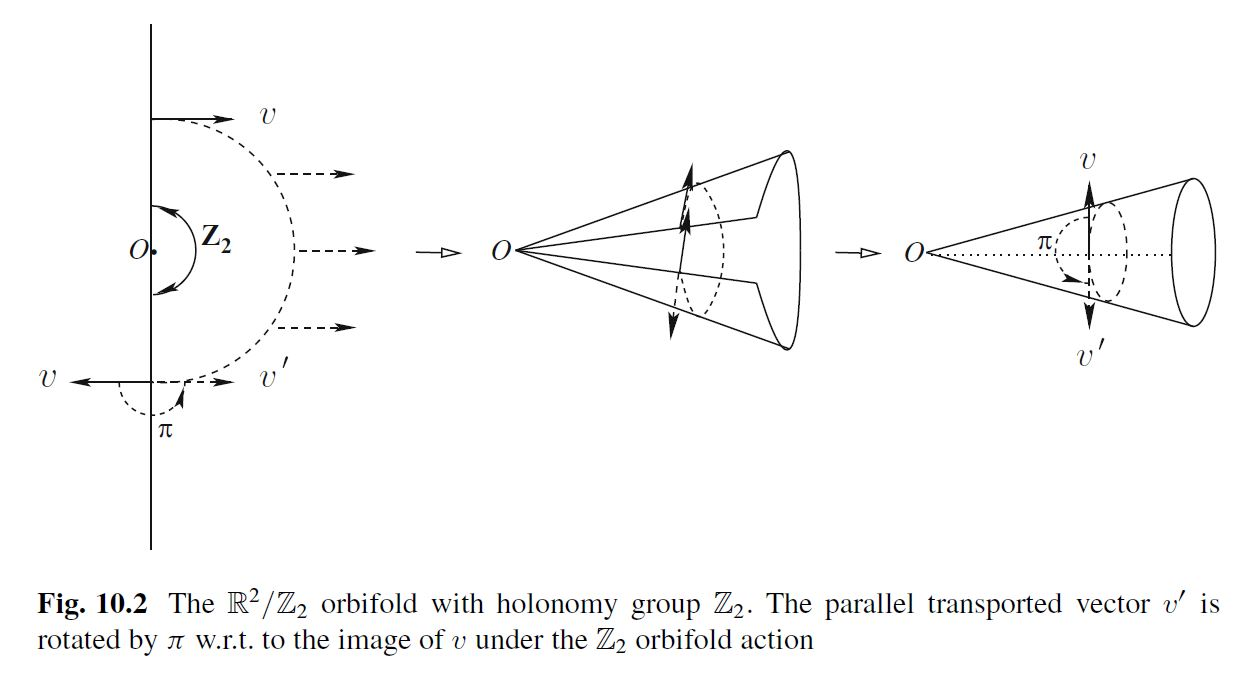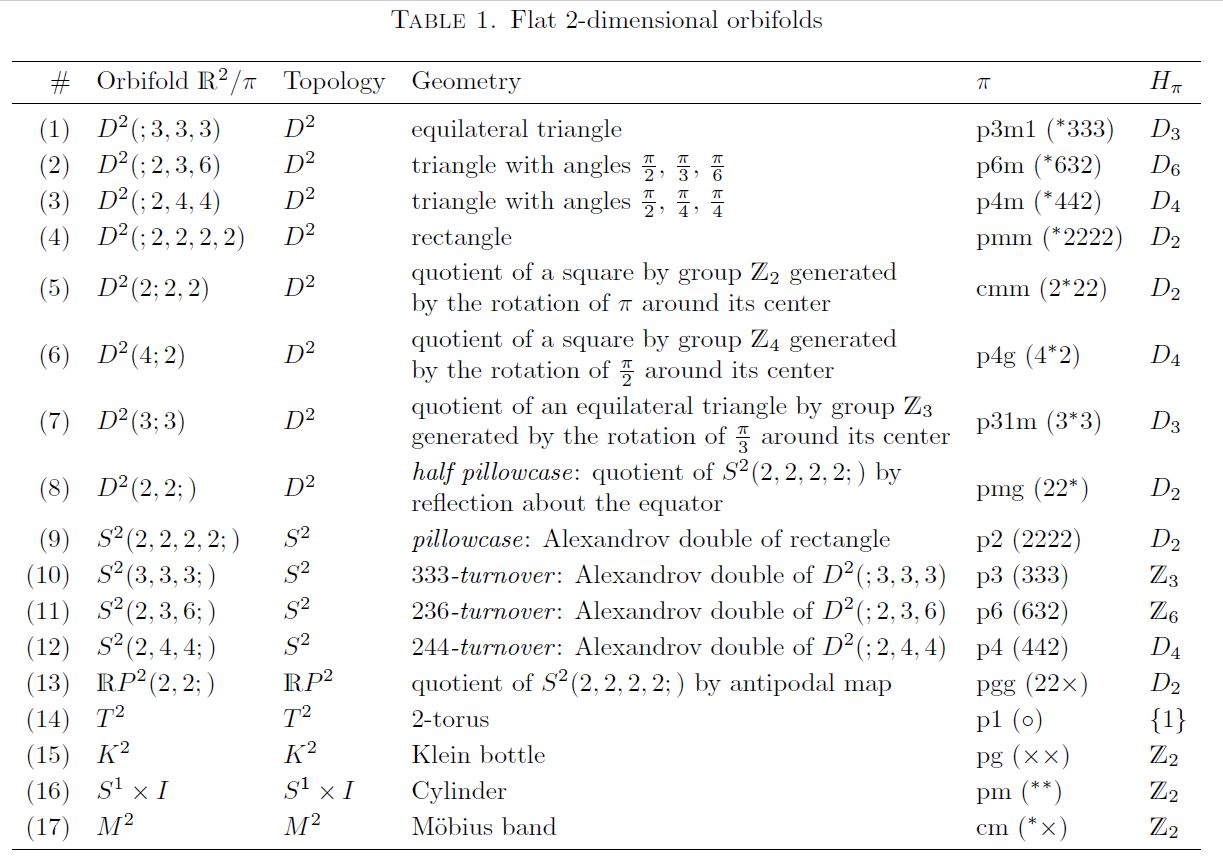# nLab Riemannian orbifold

Contents

### Context

#### Riemannian geometry

Riemannian geometry

Ingredients

Concepts

Constructions

Examples

Theorems

# Contents

## Idea

The concept of Riemannian orbifolds is the joint generalization of the concepts of Riemannian manifolds and orbifolds:

A Riemannian orbifold is an orbifold equipped with an orbifold atlas where each chart $(\widehat{U}_i, G)$ is equipped with a Riemannian metric such that the action of $G$ is by isometries, and such that the transition functions from one chart to the other are isometries.

A key aspect is that the orbifold singularities behave like carrying singular curvature, notably there are flat orbifolds (also “Euclidean orbifolds”, i.e. Riemannian orbifolds with vanishing Riemann curvature away from the singularities) whose underlying topological spaces are n-spheres (see below).

Key examples of flat orbifolds are global homotopy quotients $\mathbb{T}^n \sslash G$ of the n-torus $\mathbb{T}^n$ equipped with its canonical flat Riemannian metric. These flat orbifolds are called toroidal orbifolds.

## Properties

under construction

Every flat orbifold whose underlying metric space is connected and complete) is a global quotient of Euclidean space/Cartesian space $\mathbb{R}^n$

## Examples

### Non-compact orbifolds

Basic examples of non-compact Riemannian orbifolds are conical singularities.

In the flat case these are homotopy quotients of the form $V\sslash G$ for $G$ a finite group and $V \in RO(G)$ a finite-dimensional orthogonal linear representation of $G$.graphics grabbed from Blumenhagen-Lüst-Theisen 13

For $V = \mathbb{H}$ equipped with the canonical action of finite subgroups of SU(2) these are the ADE-singularities.

### Compact flat orbifolds from crystallographic groups

###### Example

(compact flat orbifolds from crystallographic groups)

Let $E$ be a Euclidean space and $S \subset Iso(E)$ a crystallographic group acting on it, with translational normal subgroup lattice $N \subset S$ and corresponding point group $G = S/N$.

$\array{ & 1 && 1 \\ & \downarrow && \downarrow \\ {\text{normal subgroup} \atop \text{lattice of translations}} & N &\subset& E & {\text{translation} \atop \text{group}} \\ & \big\downarrow && \big\downarrow \\ {\text{crystallographic} \atop \text{group}} & S &\subset& Iso(E) & {\text{Euclidean} \atop \text{isometry group}} \\ & \big\downarrow && \big\downarrow \\ {\text{point} \atop \text{group}} & G &\subset& O(E) & {\text{orthogonal} \atop \text{group}} \\ & \downarrow && \downarrow \\ & 1 && 1 }$

Then the action of $G$ on $E$ descends to the quotient space torus $E/N$ (this Prop.)

$\array{ E &\overset{g}{\longrightarrow}& E \\ \big\downarrow && \big\downarrow \\ E/N &\underset{g}{\longrightarrow}& E/N }$

The resulting homotopy quotient $(E/N)\sslash G$ is a compact flat orbifold.

The following is the class of special cases of Example for point group being the involution-action by reflection at a point:

###### Example

(coordinate reflection on n-torus)

Let $\mathbb{T}^d \coloneqq \mathbb{R}^d / \mathbb{Z}^d$ be the d-torus and consider the action of the cyclic group $\mathbb{Z}_2$ by canonical coordinate reflection

$\array{ \mathbb{Z}_2 \times \mathbb{T}^d &\longrightarrow& \mathbb{T}^d \\ (\sigma, \vec x) &\mapsto& - \vec x } \,.$

The resulting homotopy quotient orbifold $\mathbb{T}^d\sslash\mathbb{Z}_2$ has $2^d$ singularities/fixed points, namely the points with all coordinates in $\{0\,,\, 1/2\, \mathrm{mod} \mathbb{Z}\}$.

In applications to string theory orbifolds of the form $\mathbb{R}^{p,1} \times \mathbb{T}^d\sslash \mathbb{Z}_2$ play the role of orientifold spacetimes with $2^d$ Op-planes.

### Flat compact 2-dimensional orbifolds

In 2 dimensions the crystallographic groups are the “wallpaper groups”. Hence, as a special case of Example , the flat compact 2-dimensional orbifolds may be classified as homotopy quotients of the 2-torus by wallpaper groups (for review see e.g. Guerreiro 09):graphics grabbed from Bettiol-Derdzinski-Piccione 18

#### 2-sphere

The 2-sphere arises, in particular, as the quotient space of the torus $\mathbb{C}/\mathbb{Z}^2$ by the involution $z \mapsto -z$, exhibited by the Weierstrass elliptic function (e.g. Mukase 04, end of 1.4. This realizes the 2-sphere as the underlying coarse space of a flat orbifold with four singular points, called the pillowcase orbifold.

### Flat compact 3-dimensional orbifolds

#### 3-Sphere

Many flat compact oriented 3-dimensional orbifolds have coarse underlying topological space the 3-sphere (Dunbar 88)

### Flat compact 4-dimensional orbifolds

#### Kummer surface

The orbifold quotient of the 4-torus by the sign involution on all four canonical coordinates is the flat compact 4-dimensional orbifold known as the Kummer surface $T^4 \sslash \mathbb{Z}_2$ – the special case of Example for $d = 4$. This is a singular K3-surface (e.g. Bettiol-Derdzinski-Piccione 18, 5.5)graphics grabbed from Snowden 11

Also $\mathbb{T}^4\sslash\mathbb{Z}_4$ gives a toroidal orbifold. As orientifolds with D5-branes in type IIB string theory these are discussed in Buchel-Shiu-Tye 99, Sec. II.

#### 4-Sphere

The complex projective space $\mathbb{C}P^2$ is an orbifold quotient of the 4-torus (see this MO comment), in generalization of how the Weierstrass elliptic function exhibits the Riemann sphere as $\mathbb{T}^2/\mathbb{Z}_2$ (above).

A further $\mathbb{Z}_2$-quotient of $\mathbb{C}P^2$ gives the 4-sphere (see at 4-sphere as quotient of complex projective plane).

see FRTV 12

see G2-orbifold

### General

Real-analytic Riemannian orbifolds:

• Marja Kankaanrinta, On real analytic orbifolds and Riemannian metrics, Algebraic & Geometric Topology 13 (2013) 2369–2381 (doi:10.2140/agt.2013.13.2369)

The Yamabe invariant:

• Kazuo Akutagawal, Computations of the orbifold Yamabe invariant, Mathematische Zeitschrift volume 271, pages 611–625 (2012) (doi:10.1007/s00209-011-0880-0)

More generally, G-structures on orbifolds:

• A. V. Bagaev, N. I. Zhukova , The Automorphism Groups of Finite Type $G$-Structures on Orbifolds, Siberian Mathematical Journal 44, 213–224 (2003) (doi:10.1023/A:1022920417785)

• Robert Wolak, Orbifolds, geometric structures and foliations. Applications to harmonic maps, Rendiconti del seminario matematico - Universita politecnico di Torino, vol. 73/1 , 3-4 (2016), 173-187 (arXiv:1605.04190)

Discussion of gravity and maybe quantum gravity on orbifolds:

• Helio V. Fagundes, Teofilo Vargas, Orbifolds, Quantum Cosmology, and Nontrivial Topology (arXiv:gr-qc/0611048)

Discussion of perturbative string theory on toroidal orbifolds

For more see the references at orbifold.

### Flat orbifolds

#### Of dimension 2

In 2 dimensions

2d toroidal orientifolds:

#### Of dimension 3

• William Dunbar, Geometric orbifolds, Rev. Mat. Univ. Complutense Madr. 1, No.1-3, 67-99 (1988)

#### Of dimension 4

Flat (toroidal) orbifolds of dimension 4:

In the the context of black holes in string theory:

• Justin R. David, Gautam Mandal, Spenta R. Wadia, Microscopic Formulation of Black Holes in String Theory, Phys.Rept.369:549-686,2002 (arXiv:hep-th/0203048)

In the context of RR-field tadpole cancellation for intersecting D-brane models on toroidal orientifolds:

Specifically K3 orientifolds ($\mathbb{T}^4/G_{ADE}$) in type IIB string theory, hence for D9-branes and D5-branes:

Specifically K3 orientifolds ($\mathbb{T}^4/G_{ADE}$) in type IIA string theory, hence for D8-branes and D4-branes:

Systematic construction of Ricci flat Riemannian metrics on K3 orbifolds:

In the context of Mathieu moonshine from string sigma models on K3s:

#### Of dimension 6

In 6 dimensions (mostly motivated as singular Calabi-Yau compactifications of heterotic string theory to 4d)